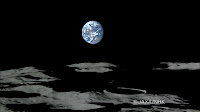Page Views on Nuit Blanche since July 2010

My papers on ArXiv:
Approximating Kernels at the speed of Light
&
Imaging with Nature

LightOn
LinkedIn (727)|| on CrunchBase || our Blog
(2452)
(3967)
(1333)||
Attendant references pages:
The Advanced Matrix Factorization Jungle Page ||

Paris Machine Learning
@Meetup.com (8016 members) || @Archives

Wednesday, November 14, 2007

Compressed Sensing: Another deterministic code, a LASSO-CS root finder and a tree based greedy algorithmIt looks like I overlooked a fifth deterministic code for measurement in Compressed Sensing. It is featured in Annihilating filter-based decoding in the compressed sensing framework by Ali Hormati and Martin Vetterli

Recent results in compressed sensing or compressive sampling suggest that a relatively small set of measurements taken as the inner product with universal random measurement vectors can well represent a source that is sparse in some fixed basis. By adapting a deterministic, non-universal and structured sensing device, this paper presents results on using the annihilating filter to decode the information taken in this new compressed sensing environment. The information is the minimum amount of nonadaptive knowledge that makes it possible to go back to the original object. We will show that for a $k$-sparse signal of dimension $n$, the proposed decoder needs $2k$ measurements and its complexity is of $O(k^2)$ whereas for the decoding based on the $\ell_1$ minimization, the number of measurements needs to be of $O(k\log(n))$ and the complexity is of $O(n^3)$. In the case of noisy measurements, we first denoise the signal using an iterative algorithm that finds the closest rank $k$ and Toeplitz matrix to the measurements matrix (in Frobenius norm) before applying the annihilating filter method. Furthermore, for a $k$-sparse vector with known equal coefficients, we propose an algebraic decoder which needs only $k$ measurements for the signal reconstruction. Finally, we provide simulation results that demonstrate the performance of our algorithm.

From the same lab, but the document is private: Efficient and Stable Acoustic Tomography Using Sparse Reconstruction Methods by Ivana Jovanovic, Ali Hormati, Luciano Sbaiz and Martin Vetterli. The abstract reads:
We study an acoustic tomography problem and propose a new inversion technique based on sparsity. Acoustic tomography observes the parameters of the medium that influence the speed of sound propagation. In the human body, the parameters that mostly influence the sound speed are temperature and density, in the ocean - temperature and current, in the atmosphere - temperature and wind. In this study, we focus on estimating temperature in the atmosphere using the information on the average sound speed along the propagation path. The latter is practically obtained from travel time measurements. We propose a reconstruction algorithm that exploits the concept of sparsity. Namely, the temperature is assumed to be a linear combination of some functions (e.g. bases or set of different bases) where many of the coefficients are known to be zero. The goal is to find the non-zero coefficients. To this end, we apply an algorithm based on linear programming that under some constrains finds the solution with minimum l0 norm. This is actually equivalent to the fact that many of the unknown coefficients are zeros. Finally, we perform numerical simulations to assess the effectiveness of our approach. The simulation results confirm the applicability of the method and demonstrate high reconstruction quality and robustness to noise.
I also found the presentation of this paper from Ewout van den Berg and Michael Friedlander entitled " In pursuit of a root". The presentation is much clearer about what they are talking about ( I am a visual person). In essence they try to solve a Basis Pursuit problem by a Lasso and rely on the fact that the correspondence between the two problems is continuous and differentiable. Very nice.Similarly, this presentation-talk by Minh Do entitled "Practical Compressed Sensing" puts a new light on the possible extension of compressed sensing. Namely the fact that not only most signals are sparse in a good basis of representation but most coefficients of importance lie in a tree across different scalesMinh Do has already published a scheme based on this observation called: Tree-Based Orthogonal Matching Pursuit Algorithm For Signal Reconstruction with Chinh La. I am curious on how the implementation will fare in comparison to other reconstruction techniques.

Photo Credit: JAXA, Japanese probe Kayuga transmitted this HDTV of an Earth rise above the Moon
at 2:52 p.m. on November 7, 2007 (JST).The "Earth-rise" movie is here, and the "Earth-set" movie is here.